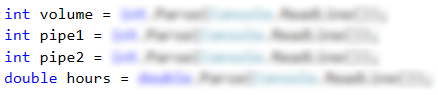# Problem: Pipes in Pool

A pool with volume V fills up via two pipes. Each pipe has a certain flow rate (the liters of water, flowing through a pipe for an hour). A worker starts the pipes simultaneously and goes out for N hours. Write a program that finds the state of the pool the moment the worker comes back.

## Input Data

Four lines are read from the console:

• The first line contains a number V – the volume of the pool in liters – an integer in the range of [1 … 10000].
• The second line contains a number P1 – the flow rate of the first pipe per hour – an integer in the range of [1 … 5000].
• The third line contains a number P2 – the flow rate of the second pipe per hour – an integer in the range of [1 … 5000].
• The fourth line contains a number H – the hours that the worker is absent – a floating-point number in the range of [1.0 … 24.00].

## Output Data

Print on the console one of the two possible states:

• To what extent the pool has filled up and how many percent each pipe has contributed with. All percent values must be formatted to an integer (without rounding).
• "The pool is [x]% full. Pipe 1: [y]%. Pipe 2: [z]%."
• If the pool has overflown – with how many liters it has overflown for the given time – a floating-point number.
• "For [x] hours the pool overflows with [y] liters."

Have in mind that due to the rounding to an integer, there is data loss and it is normal the sum of the percents to be 99%, not 100%.

## Sample Input and Output

Input Output Input Output
1000
100
120
3
The pool is 66% full. Pipe 1: 45%. Pipe2: 54%. 100
100
100
2.5
For 2.5 hours the pool overflows with 400 liters.

## Hints and Guidelines

In order to solve the task, we read the input data, write a few conditional statements, do some calculations and print the result.

### Processing the Input Data

From the task requirements we note that our program must have four lines from which we read the input data. The first three consist of integers and that is why the variables that will store their values will be of int type. We know that the fourth line will be a floating-point number, therefore, the variable we use will be of double type.Out next step is to declare and initialize a variable in which we are going to calculate with how many liters the pool has filled up for the time the worker was absent. We do the calculations by summing the values of the flow rates of the two pipes and multiplying them by the hours that are given as input data.### Checking the Conditions and Processing Output Data

After we have the value of the quantity of water that has flown through the pipes, the next step is to compare that quantity with the volume of the pool itself.

We do that with a simple if-else statement, where the condition will be whether the quantity of water is less than the volume of the pool. If the statement returns true, we have to print one line that contains the ratio between the quantity of water that has flown through the pipes and the volume of the pool, as well as the ratio of the quantity of the water from each pipe to the volume of the pool.

The ratio has to be in percentage, that is why all the calculations so far will be multiplied by 100. The values will be printed using placeholders, and as there is a condition the result in percentage to be formatted to two digits after the decimal point without rounding, we will use the method Math.Truncate(…).However, if the condition returns false, that means that the quantity of water is more than the volume of the pool, therefore, it has overflown. Again, the output data has to be on one line, but this time it should contain only two values – the one of the hours when the worker was absent, and the quantity of water, which is the difference between the incoming water and the volume of the pool.

## Testing in the Judge System

Test your solution here: https://judge.softuni.org/Contests/Practice/Index/507#1.